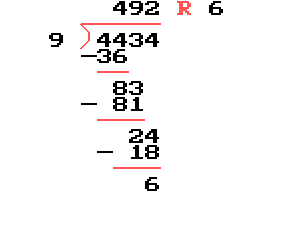# How do you do this problem

• jim1174

#### jim1174

I got this problem off the internet and need some help understanding how to do it. first you do 9 goes into 44 4 times so you put a 4 on top then you do 9 times 4 equals 36 so you put the 36 under the 44. next you do 44 minus 36 which is 8 and bring down the 3. can you please explain how to get the other numbers in the problem ?If I tell you that 9 goes into 83 9 times, can you take it the rest of the way?

ok so where does the 2 on the top come from ?

9 x 9 = 81, subtract that from the 83, gives you 2, bring down the 4 -----
Starting to see the pattern?

yes i understand that part but how do you get the 2 on the top next to the 49

9 goes into 24 2 times

9*2= 18< 24< 27= 9*3

Since "9*3" is too large, we take "2" leaving a remainder of 24- 18= 6.